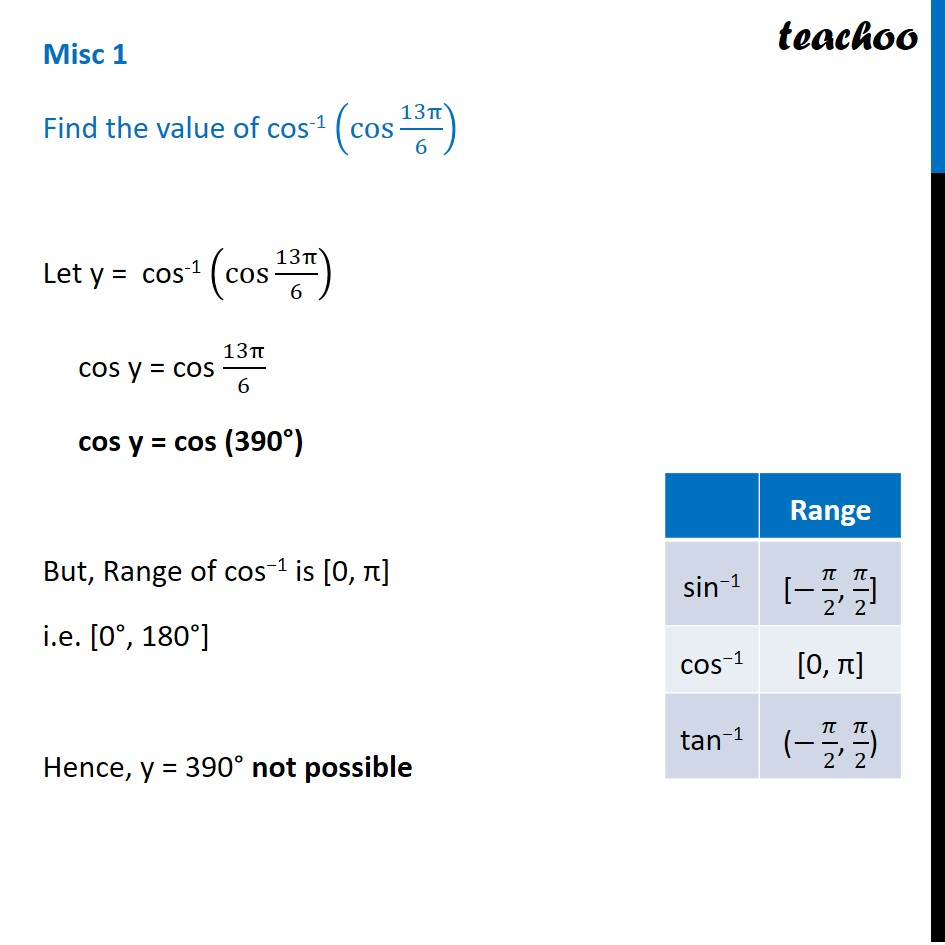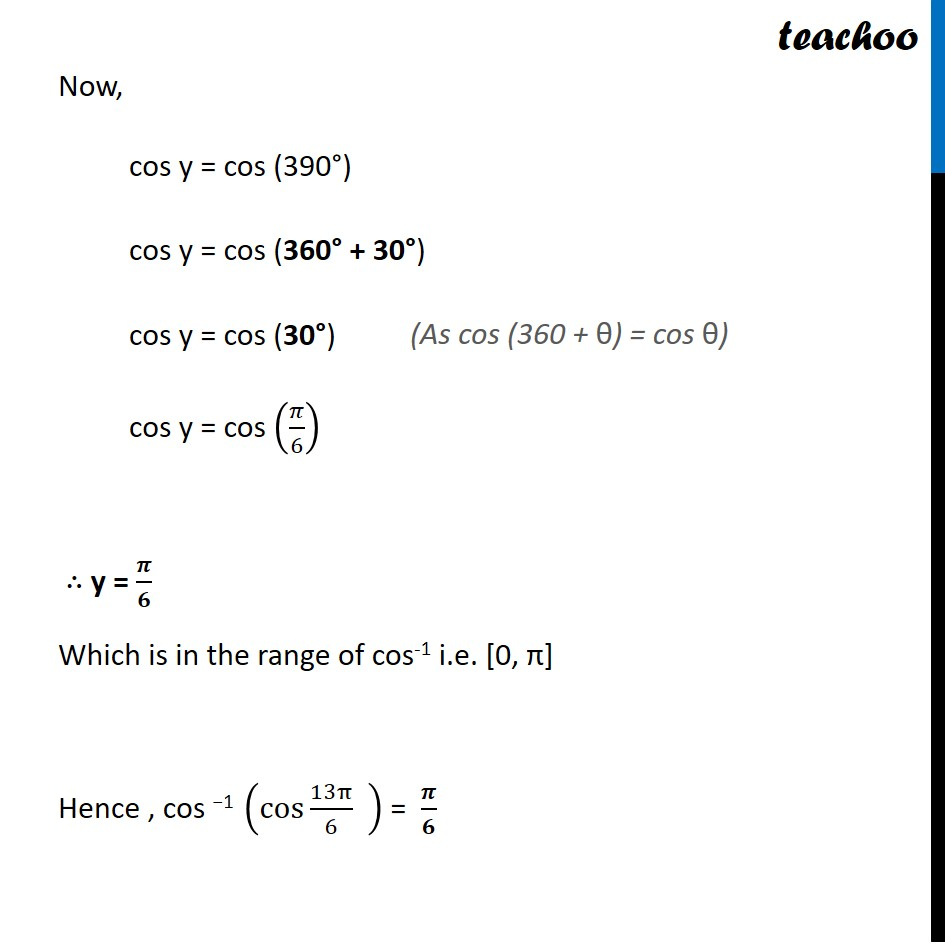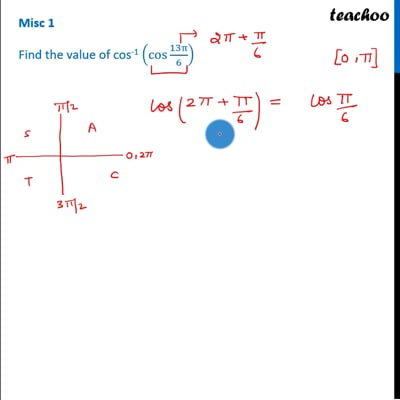Miscellaneous

Chapter 2 Class 12 Inverse Trigonometric Functions
Serial order wiseThis video is only available for Teachoo black users

Solve all your doubts with Teachoo Black (new monthly pack available now!)

### Transcript

Misc 1 Find the value of cos-1 (cos⁡〖13π/6〗 ) Let y = cos-1 (cos⁡〖13π/6〗 ) cos y = cos 13π/6 cos y = cos (390°) But, Range of cos−1 is [0, π] i.e. [0°, 180°] Hence, y = 390° not possible Now, cos y = cos (390°) cos y = cos (360° + 30°) cos y = cos (30°) cos y = cos (𝜋/6) ∴ y = 𝝅/𝟔 Which is in the range of cos-1 i.e. [0, π] Hence , cos −1 (cos 13π/6 " " ) = 𝝅/𝟔 (As cos (360 + θ) = cos θ)